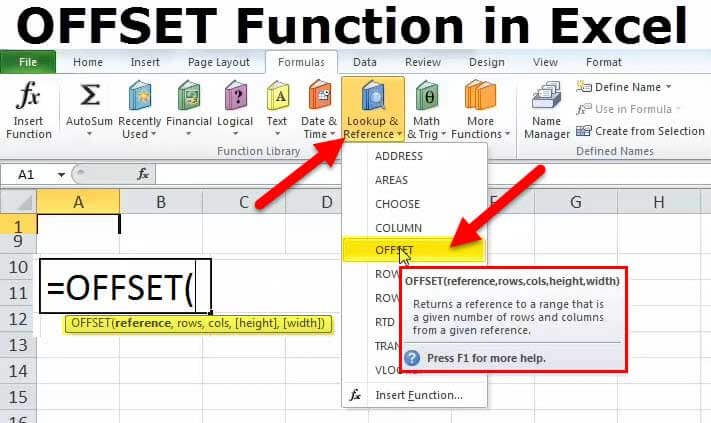# How To Use The Offset VBA In Excel

Oct 18, 2020 • edited Oct 21, 2020The Offset property in Excel VBA takes the range which is a particular number of rows and columns away from a certain range (border below for illustration only).

Place a command button on your worksheet and add the following code lines:

Dim example As Range Set example = Range(“A1:A2”) example.Offset(3, 2).Select

Result when you click the command button on the sheet:Explanation: these code lines select the range which is 3 rows below and 2 columns to the right of Range(“A1:A2”). The Offset property always takes the top left cell of a range as the starting point.

For a practical example of the offset property, see our example program Create a Pattern.

#Tutorial#How To#VBA#Range Object

How To Use Randomly Sort Data In Excel

How To Get The Size Of An Array In Excel